# Selina solutions for Concise Mathematics Class 8 ICSE chapter 16 - Understanding Shapes [Latest edition]

#### Chapters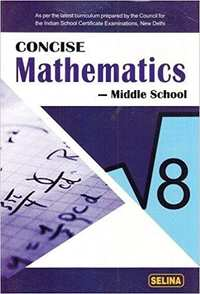## Chapter 16: Understanding Shapes

Exercise 16 (A)Exercise 16 (B)Exercise 16 (C)
Exercise 16 (A) [Pages 180 - 181]

### Selina solutions for Concise Mathematics Class 8 ICSE Chapter 16 Understanding Shapes Exercise 16 (A) [Pages 180 - 181]

Exercise 16 (A) | Q 1 | Page 180

State which of the following are polygons :

(i)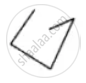(ii)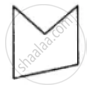(iii)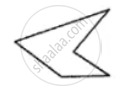(iv)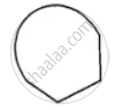(v)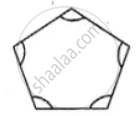(vi)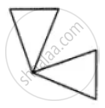If the given figure is a polygon, name it as convex or concave.

Exercise 16 (A) | Q 2.1 | Page 180

Calculate the sum of angle of a polygon with: 10 sides

Exercise 16 (A) | Q 2.2 | Page 180

Calculate the sum of angle of a polygon with: 12 sides

Exercise 16 (A) | Q 2.3 | Page 180

Calculate the sum of angle of a polygon with: 20 sides

Exercise 16 (A) | Q 2.4 | Page 180

Calculate the sum of angle of a polygon with : 25 sides

Exercise 16 (A) | Q 3.1 | Page 180

Find the number of sides in a polygon if the sum of its interior angle is: 900°

Exercise 16 (A) | Q 3.2 | Page 180

Find the number of sides in a polygon if the sum of its interior angle is: 1620°

Exercise 16 (A) | Q 3.3 | Page 180

Find the number of sides in a polygon if the sum of its interior angle is: 16 right-angles.

Exercise 16 (A) | Q 3.4 | Page 180

Find the number of sides in a polygon if the sum of its interior angle is: 32 right-angles.

Exercise 16 (A) | Q 4.1 | Page 180

Is it possible to have a polygon, whose sum of interior angle is: 870°

Exercise 16 (A) | Q 4.2 | Page 180

Is it possible to have a polygon, whose sum of interior angle is: 2340°

Exercise 16 (A) | Q 4.3 | Page 180

Is it possible to have a polygon, whose sum of interior angle is: 7 right-angles

Exercise 16 (A) | Q 4.4 | Page 180

Is it possible to have a polygon, whose sum of interior angle is:  4500°

Exercise 16 (A) | Q 5.1 | Page 180

If all the angles of a hexagon are equal, find the measure of each angle.

Exercise 16 (A) | Q 5.2 | Page 181

If all the angles of a 14-sided figure are equal ; find the measure of each angle.

Exercise 16 (A) | Q 6.1 | Page 181

Find the sum of exterior angle obtained on producing, in order, the side of a polygon with : 7 sides

Exercise 16 (A) | Q 6.2 | Page 181

Find the sum of exterior angle obtained on producing, in order, the side of a polygon with : 10 sides

Exercise 16 (A) | Q 6.3 | Page 181

Find the sum of exterior angle obtained on producing, in order, the side of a polygon with: 250 sides

Exercise 16 (A) | Q 7 | Page 181

The sides of a hexagon are produced in order. If the measures of exterior angles so obtained are (6x – 1)°, (10x + 2)°, (8x + 2)° (9x – 3)°, (5x + 4)° and (12x + 6)° ; find each exterior angle.

Exercise 16 (A) | Q 8 | Page 181

The interior angles of a pentagon are in the ratio 4 : 5 : 6 : 7 : 5. Find each angle of the pentagon.

Exercise 16 (A) | Q 9 | Page 181

Two angles of a hexagon are 120° and 160°. If the remaining four angles are equal, find each equal angle.

Exercise 16 (A) | Q 10 | Page 181

The figure, given below, shows a pentagon ABCDE with sides AB and ED parallel to each other, and ∠B : ∠C : ∠D = 5: 6: 7.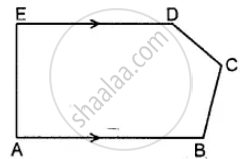(i) Using the formula, find the sum of the interior angles of the pentagon.

(ii) Write the value of ∠A + ∠E

(iii) Find angles B, C and D.

Exercise 16 (A) | Q 11 | Page 181

Two angles of a polygon are right angles and the remaining are 120° each. Find the number of sides in it.

Exercise 16 (A) | Q 12 | Page 181

In a hexagon ABCDEF, side AB is parallel to side FE and ∠B : ∠C : ∠D : ∠E = 6 : 4 : 2 : 3. Find ∠B and ∠D.

Exercise 16 (A) | Q 13 | Page 181

The angles of a hexagon are x + 10°, 2x + 20°, 2x – 20°, 3x – 50°, x + 40° and x + 20°. Find x.

Exercise 16 (A) | Q 14 | Page 181

In a pentagon, two angles are 40° and 60°, and the rest are in the ratio 1 : 3 : 7. Find the biggest angle of the pentagon.

Exercise 16 (B) [Pages 184 - 185]

### Selina solutions for Concise Mathematics Class 8 ICSE Chapter 16 Understanding Shapes Exercise 16 (B) [Pages 184 - 185]

Exercise 16 (B) | Q 1 | Page 184

Fill in the blanks :
In case of regular polygon, with :

 No.of.sides Each exterior angle Each interior angle (i) ___8___ _______ ______ (ii) ___12____ _______ ______ (iii) _________ _____72°_____ ______ (iv)  _________ _____45°_____ ______ (v)  _________ __________ _____150°_____ (vi) ________ __________ ______140°____
Exercise 16 (B) | Q 2.1 | Page 184

Find the number of sides in a regular polygon, if its interior angle is: 160°

Exercise 16 (B) | Q 2.2 | Page 184

Find the number of sides in a regular polygon, if its interior angle is: 135°

Exercise 16 (B) | Q 2.3 | Page 184

Find the number of sides in a regular polygon, if its interior angle is: 1 1/5 of a right angle

Exercise 16 (B) | Q 3.1 | Page 184

Find the number of sides in a regular polygon, if its exterior angle is : 1/3 of right angle

Exercise 16 (B) | Q 3.2 | Page 184

Find the number of sides in a regular polygon, if its exterior angle is: two-fifth of right angle

Exercise 16 (B) | Q 4.1 | Page 184

Is it possible to have a regular polygon whose interior angle is : 170°

Exercise 16 (B) | Q 4.2 | Page 184

Is it possible to have a regular polygon whose interior angle is:

138°

Exercise 16 (B) | Q 5.1 | Page 184

Is it possible to have a regular polygon whose each exterior angle is: 80°

Exercise 16 (B) | Q 5.2 | Page 184

Is it possible to have a regular polygon whose each exterior angle is: 40° of a right angle.

Exercise 16 (B) | Q 6 | Page 184

Find the number of sides in a regular polygon, if its interior angle is equal to its exterior angle.

Exercise 16 (B) | Q 7 | Page 184

The exterior angle of a regular polygon is one-third of its interior angle. Find the number of sides in the polygon.

Exercise 16 (B) | Q 8 | Page 184

The measure of each interior angle of a regular polygon is five times the measure of its exterior angle. Find :

(i) measure of each interior angle ;
(ii) measure of each exterior angle and
(iii) number of sides in the polygon.

Exercise 16 (B) | Q 9 | Page 184

The ratio between the interior angle and the exterior angle of a regular polygon is 2: 1. Find:

(i) each exterior angle of the polygon ;
(ii) number of sides in the polygon.

Exercise 16 (B) | Q 10 | Page 184

The ratio between the exterior angle and the interior angle of a regular polygon is 1 : 4. Find the number of sides in the polygon.

Exercise 16 (B) | Q 11 | Page 184

The sum of interior angles of a regular polygon is twice the sum of its exterior angles. Find the number of sides of the polygon.

Exercise 16 (B) | Q 12 | Page 184

AB, BC and CD are three consecutive sides of a regular polygon. If angle BAC = 20° ; find :

(i) its each interior angle,
(ii) its each exterior angle
(iii) the number of sides in the polygon.

Exercise 16 (B) | Q 13 | Page 184

Two alternate sides of a regular polygon, when produced, meet at the right angle. Calculate the number of sides in the polygon.

Exercise 16 (B) | Q 14 | Page 184

In a regular pentagon ABCDE, draw a diagonal BE and then find the measure of:

(i) ∠BAE
(ii) ∠ABE
(iii) ∠BED

Exercise 16 (B) | Q 15 | Page 184

The difference between the exterior angles of two regular polygons, having the sides equal to (n – 1) and (n + 1) is 9°. Find the value of n.

Exercise 16 (B) | Q 16 | Page 184

If the difference between the exterior angle of a 'n' sided regular polygon and an (n + 1) sided regular polygon is 12°, find the value of n.

Exercise 16 (B) | Q 17 | Page 184

The ratio between the number of sides of two regular polygons is 3 : 4 and the ratio between the sum of their interior angles is 2 : 3. Find the number of sides in each polygon.

Exercise 16 (B) | Q 18 | Page 184

Three of the exterior angles of a hexagon are 40°, 51 ° and 86°. If each of the remaining exterior angles is x°, find the value of x.

Exercise 16 (B) | Q 19.1 | Page 184

Calculate the number of sides of a regular polygon, if:  its interior angle is five times its exterior angle.

Exercise 16 (B) | Q 19.2 | Page 185

Calculate the number of sides of a regular polygon, if: the ratio between its exterior angle and interior angle is 2: 7.

Exercise 16 (B) | Q 19.3 | Page 185

Calculate the number of sides of a regular polygon, if: its exterior angle exceeds its interior angle by 60°.

Exercise 16 (B) | Q 20 | Page 185

The sum of interior angles of a regular polygon is thrice the sum of its exterior angles. Find the number of sides in the polygon.

Exercise 16 (C) [Pages 187 - 188]

### Selina solutions for Concise Mathematics Class 8 ICSE Chapter 16 Understanding Shapes Exercise 16 (C) [Pages 187 - 188]

Exercise 16 (C) | Q 1 | Page 187

Two angles of a quadrilateral are 89° and 113°. If the other two angles are equal; find the equal angles.

Exercise 16 (C) | Q 2 | Page 187

Two angles of a quadrilateral are 68° and 76°. If the other two angles are in the ratio 5 : 7; find the measure of each of them.

Exercise 16 (C) | Q 3 | Page 187

Angles of a quadrilateral are (4x)°, 5(x+2)°, (7x – 20)° and 6(x+3)°. Find :

(i) the value of x.
(ii) each angle of the quadrilateral.

Exercise 16 (C) | Q 4 | Page 187

Use the information given in the following figure to find :

(i) x
(ii) ∠B and ∠C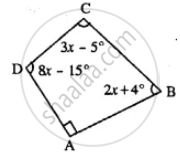Exercise 16 (C) | Q 5 | Page 187

In quadrilateral ABCD, side AB is parallel to side DC. If ∠A : ∠D = 1 : 2 and ∠C : ∠B = 4 : 5

(i) Calculate each angle of the quadrilateral.

(ii) Assign a special name to quadrilateral ABCD

Exercise 16 (C) | Q 6 | Page 187

From the following figure find ;
(i) x
(ii) ∠ABC
(iii) ∠ACD

Exercise 16 (C) | Q 7 | Page 187

Given : In quadrilateral ABCD ; ∠C = 64°, ∠D = ∠C – 8° ; ∠A = 5(a+2)° and ∠B = 2(2a+7)°.
Calculate ∠A.

Exercise 16 (C) | Q 8 | Page 187

In the given figure : ∠b = 2a + 15 and ∠c = 3a + 5; find the values of b and c.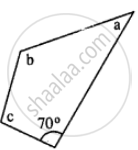Exercise 16 (C) | Q 9 | Page 187

Three angles of a quadrilateral are equal. If the fourth angle is 69°; find the measure of equal angles.

Exercise 16 (C) | Q 10 | Page 187

In quadrilateral PQRS, ∠P : ∠Q : ∠R : ∠S = 3 : 4 : 6 : 7.
Calculate each angle of the quadrilateral and then prove that PQ and SR are parallel to each other
(i) Is PS also parallel to QR?
(ii) Assign a special name to quadrilateral PQRS.

Exercise 16 (C) | Q 11 | Page 187

Use the information given in the following figure to find the value of x.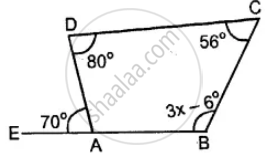Exercise 16 (C) | Q 12 | Page 187

The following figure shows a quadrilateral in which sides AB and DC are parallel. If ∠A : ∠D = 4 : 5, ∠B = (3x – 15)° and ∠C = (4x + 20)°, find each angle of the quadrilateral ABCD.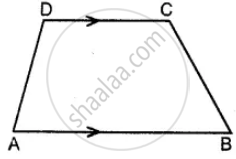Exercise 16 (C) | Q 13 | Page 188

Use the following figure to find the value of x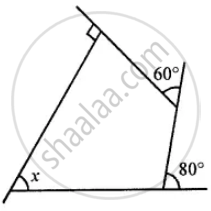Exercise 16 (C) | Q 14 | Page 188

ABCDE is a regular pentagon. The bisector of angle A of the pentagon meets the side CD in point M. Show that ∠AMC = 90°.

Exercise 16 (C) | Q 15 | Page 188

In a quadrilateral ABCD, AO and BO are bisectors of angle A and angle B respectively. Show that:

∠AOB = 1/2 (∠C + ∠D)

## Chapter 16: Understanding Shapes

Exercise 16 (A)Exercise 16 (B)Exercise 16 (C)## Selina solutions for Concise Mathematics Class 8 ICSE chapter 16 - Understanding Shapes

Selina solutions for Concise Mathematics Class 8 ICSE chapter 16 (Understanding Shapes) include all questions with solution and detail explanation. This will clear students doubts about any question and improve application skills while preparing for board exams. The detailed, step-by-step solutions will help you understand the concepts better and clear your confusions, if any. Shaalaa.com has the CISCE Concise Mathematics Class 8 ICSE solutions in a manner that help students grasp basic concepts better and faster.

Further, we at Shaalaa.com provide such solutions so that students can prepare for written exams. Selina textbook solutions can be a core help for self-study and acts as a perfect self-help guidance for students.

Concepts covered in Concise Mathematics Class 8 ICSE chapter 16 Understanding Shapes are Different Types of Curves - Closed Curve, Open Curve, Simple Curve., Concept of Polygone, Sum of Angles of a Polynomial, Sum of Exterior Angles of a Polynomial, Regular Polynomial, Concept of Quadrilaterals - Sides, Adjacent Sides, Opposite Sides, Angle, Adjacent Angles and Opposite Angles.

Using Selina Class 8 solutions Understanding Shapes exercise by students are an easy way to prepare for the exams, as they involve solutions arranged chapter-wise also page wise. The questions involved in Selina Solutions are important questions that can be asked in the final exam. Maximum students of CISCE Class 8 prefer Selina Textbook Solutions to score more in exam.

Get the free view of chapter 16 Understanding Shapes Class 8 extra questions for Concise Mathematics Class 8 ICSE and can use Shaalaa.com to keep it handy for your exam preparation